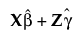Fitting Linear Models > Mixed Models > Conditional Model Inference > Actual by Conditional Predicted Plot
Publication date: 04/12/2021

##Actual by Conditional Predicted Plot

The Actual by Conditional Predicted plot appears by default. It provides a visual assessment of model fit that accounts for variation due to random effects. It plots the observed values of Y against the conditional predicted values of Y. These are the predicted values obtained if you select Save Columns > Conditional Prediction Formula.

Denote the linear mixed model by E[Y|γ] = Xβ + Zγ. Here β is the vector of fixed effect coefficients and γ is the vector of random effect coefficients. The conditional predictions are the predictions obtained from the model given by.

Want more information? Have questions? Get answers in the JMP User Community (community.jmp.com).